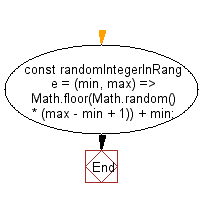# JavaScript: Get a random integer in the specified range

## JavaScript fundamental (ES6 Syntax): Exercise-34 with Solution

Write a JavaScript program to get a random integer in the specified range.

• Use Math.random() to generate a random number and map it to the desired range.
• Use Math.floor() to make it an integer.

Sample Solution:

JavaScript Code:

``````//#Source https://bit.ly/2neWfJ2
const randomIntegerInRange = (min, max) => Math.floor(Math.random() * (max - min + 1)) + min;
console.log(randomIntegerInRange(0, 5));
console.log(randomIntegerInRange(2, 5));
console.log(randomIntegerInRange(5, -5));
console.log(randomIntegerInRange(-2, -7));
```
```

Sample Output:

```1
3
-1
-4
```

Pictorial Presentation:Flowchart:Live Demo:

See the Pen javascript-basic-exercise-1-34 by w3resource (@w3resource) on CodePen.

Improve this sample solution and post your code through Disqus

What is the difficulty level of this exercise?

Test your Programming skills with w3resource's quiz.

﻿

## JavaScript: Tips of the Day

Returns an array of n-tuples of consecutive elements

Example:

```const tips_arr = (n, arr) =>  n > arr.length ? [] : arr.slice(n - 1).map((v, i) =>[...arr.slice(i, i + n - 1), v]);
console.log(tips_arr(2, [1, 2, 3, 4, 5]));
console.log(tips_arr(3, [1, 2, 3, 4, 5]));
console.log(tips_arr(5, [1, 2, 3, 4]));
```

Output:

```[[1, 2], [2, 3], [3, 4], [4, 5]]
[[1, 2, 3], [2, 3, 4], [3, 4, 5]]
[]
```